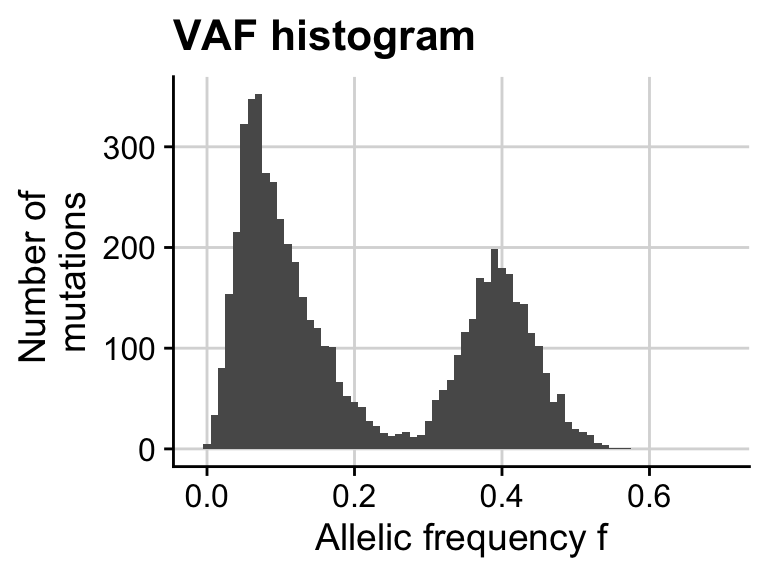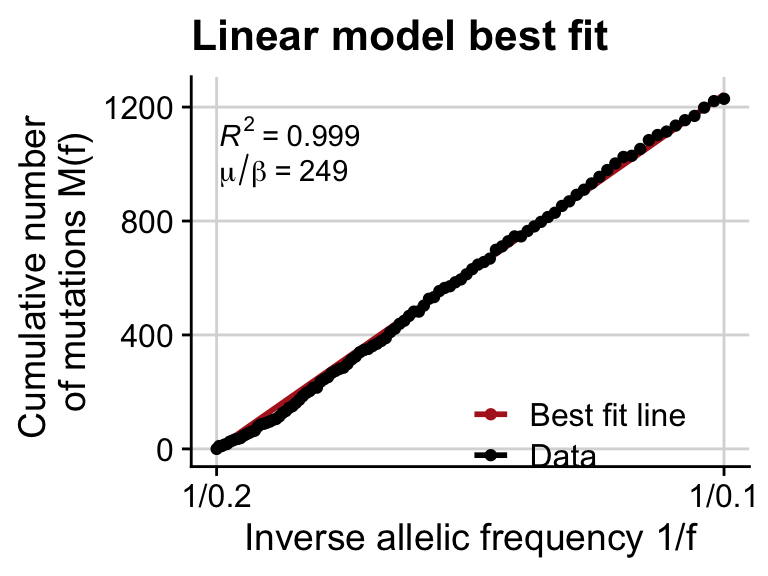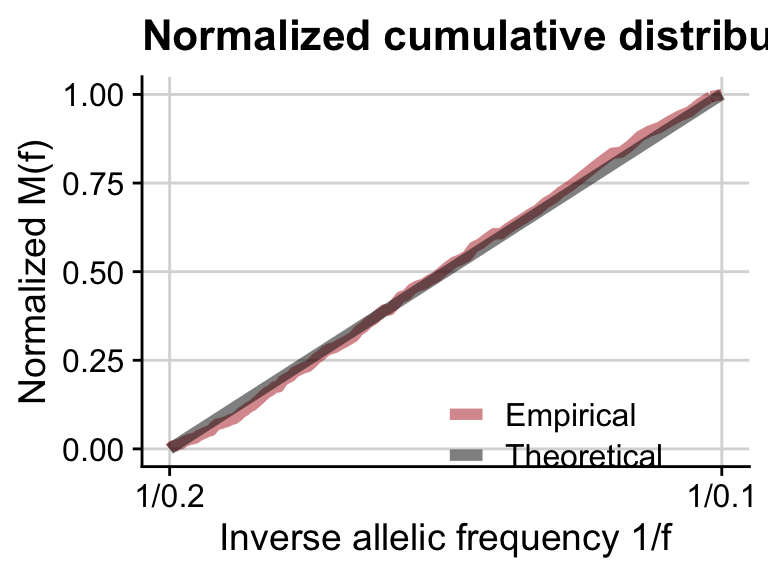## Load package

After you have installed the package, you can load the package via the normal command. This will also load some test data.

library(neutralitytestr)

The test data files include 2 simulated VAF distributions, one under a neutral evolutionary model and one with a non neutral evolutionary model. The test data are vectors of variant allele frequency, and are named VAFselection and VAFneutral. First of all we print the first few elements of one of these vectors.

head(VAFselection)
##  0.3669725 0.4056604 0.4226804 0.3333333 0.4200000 0.3700000

The test data were generated using an evolutionary model of cancer which produces synthetic sequencing data. The test data here were generated to approximately mimic whole genome sequencing data. There are $$\sim 5000$$ mutations in both datasets and the data was “sequenced” to 100X. Also included was normal contamination of 20%, that is why we observe the peak at roughly 0.4 ($$2 \times 0.4 = 0.8$$) which represents the clonal mutations in the sample, that is mutations present in every cancer cell. For both data the effective mutation rate (or equivalently the per tumour doubling mutation rate) is 200 corresponding to a WGS base per rate of $$\sim 7 \times 10^{-8}$$. For the VAFneutral data a neutral model where all cells have the same fitness was used, for the VAFselection data there is a single subclone at frequency 0.44 hence the peak at around 0.2 in the VAF spectrum (as will be seen below).

## neutralitytest object

The basic functionality of the neutralitytestr package is achieved by creating a neutralitytest object. The neutralitytest object contains a range of metrics to test for neutrality, and makes plotting histograms and cumulative distributions to visualize the output easy. The neutralitytest function takes a vector of VAFs and an upper and lower limit for the frequency range over which we wish to test whether the data is consistent with a neutral model, and then calculates all 4 metrics.

s <- neutralitytest(VAFselection, fmin = 0.1, fmax = 0.25)
## This package has been superseded by MOBSTER, see Caravagna et al. Nature Genetics 2020.

We can the print a summary of the neutralitytest object for the synthetic data with selection. This prints out all values and associated p-values for all the metrics. The p-values are the p-values under the null model of neutral evolution.

summary(s)
## Summary of neutrality metrics:
##
## Area:
##   value =  0.2029819 , p-value =  0.007
## Kolmogorov Distance:
##   value =  0.3357769 , p-value =  0.008
## Mean distance:
##   value =  0.202308 , p-value =  0.007
## R^2:
##   value =  0.9373371 , p-value =  0.009
##
## Effective mutation rate =  462.9867

Rather than manually inputting the minimum and maximum of the integration range we can also input values for the read depth ($$D$$), the cellularity (% tumour content, c) of the sample, overdispersion of the sequencing data (rho) and the ploidy ($$\pi$$). This will calculate the expected standard deviation of the clonal cluster using the following equation: $SD = \sqrt{\frac{1 + (D-1)\times\rho}{D}}$ We expect the mean of the cluster to be at the following frequency: $M = \frac{c}{\pi}$ Then the the maximum of the integration range, fmax will be set to $$M - 2\times SD$$.

We’ll now test the model on some synthetic data from a neutral evolutionary model using by inputting values for the read depth, cellularity, overdispersion parameter rho and ploidy and allowing the limits to be calculated automatically.

n <- neutralitytest(VAFneutral, read_depth = 100.0, cellularity = 0.8, rho = 0.0, ploidy = 2)
## This package has been superseded by MOBSTER, see Caravagna et al. Nature Genetics 2020.
## Using inputted read depth to calculate integration range
##  Standard deviation of clonal peak is calculated to be 0.1
##  Mean of peak is 0.4.
##  Using integration range - (0.1,0.2)
summary(n)
## Summary of neutrality metrics:
##
## Area:
##   value =  0.003116455 , p-value =  0.967
## Kolmogorov Distance:
##   value =  0.04182846 , p-value =  0.962
## Mean distance:
##   value =  0.01438067 , p-value =  0.96
## R^2:
##   value =  0.999 , p-value =  0.952
##
## Effective mutation rate =  248.8715

## Plotting

The neutralitytest object makes plotting simple. Plotting uses ggplot, so plots can easily modified using the usual ggplot syntax. First of all we can plot a histogram of the VAFs.

vaf_histogram(n)We can also plot the cumulative distribution along with the least squares best fit line, from which we get the $$R^2$$ value and the estimated mutation rate.

lsq_plot(n)And finally the normalized cumulative distribution, which is used to calculate the kolmogorov distance and the area metrics.

normalized_plot(n)Invoking the plot command will plot all 3 plots together, all these plots are generated using ggplot2, they can therefore be modified as any other ggplot object would be including saving etc.

gout <- plot_all(s)
gout